## Example Questions

### Example Question #7 : Decimals

What is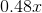equivalent to?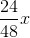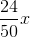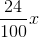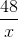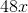Explanation:

We need to convert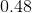into a fraction.

To do this, write down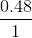Now, because the decimal goes to the hundreth place, multiply the numerator and denominator of the fraction by 100.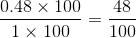Now, simplify the fraction.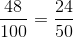### Example Question #2 : Decimals With Fractions

A tub of food containspounds of vegetables,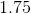pounds of lard, andpounds of sausage.  What is its total weight as an improper fraction?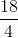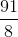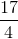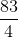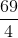Explanation:

To begin with, it is easiest just to add these decimals together using your calculator: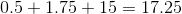Now, this is the same thing as: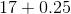We can rewrite this: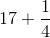To find this, you need to give the two numbers a common denominator: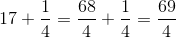### Example Question #2 : How To Find The Fractional Equivalent Of A Decimal

What is the fractional equivalent of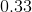?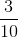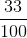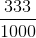Explanation:

In decimal formis said 33 hundredths.

This is equal to.

This fraction cannot be reduced any further therefore it is in its final answer form.

### Example Question #2 : Decimals

Write 0.45 as a fraction.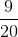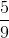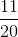Explanation:

.45 is equivalent to 45 out of 100, or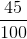.

Divide both the numerator and denominator by 5 to simplify the fraction:### Example Question #1 : How To Find The Fractional Equivalent Of A Decimal

Convert this decimal to a fraction in reduced form: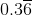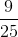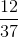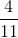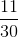Explanation:

To figure out what fraction this decimal corresponds to, we have to get rid of the repeating part, otherwise we would be dealing with an infinite recursion and we don't have the tools to deal with that yet. Instead, we will set the decimal equal toand multiply it by 10, and also multiply it by 100.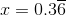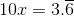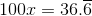Notice that in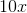and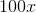we have the same repeating decimal part. So if we subtract them, it disappears: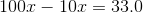Now all that remains is to do the algebra.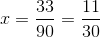is our final answer.

### Example Question #2 : How To Find The Fractional Equivalent Of A Decimal

What is the fractional equivalent of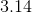?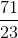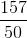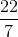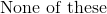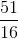Explanation:

To find the fractional equivalent of a decimal, set the decimal as a fraction over, then multiply top and bottom by one power offor each digit after the decimal. In this case, we have two digits after the decimal, so we multiply top and bottom by: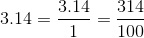Now, start simplifying.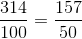Since we cannot reduce further,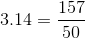.